Home > CC3 > Chapter 9 > Lesson 9.2.3 > Problem9-88

9-88.
1. Find the missing length or area. Homework Help ✎

1.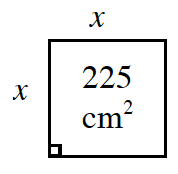2.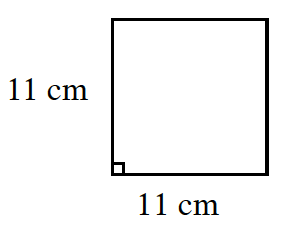3.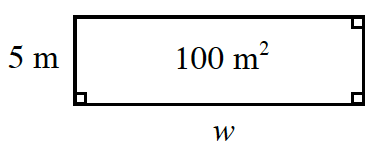4.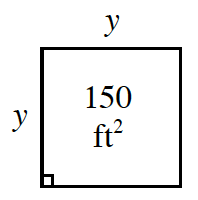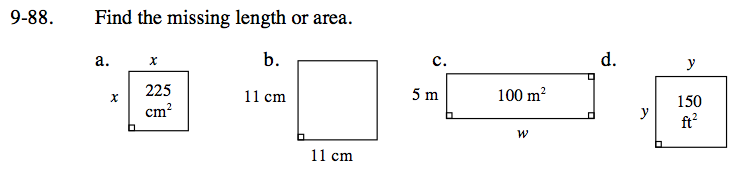You can identify this figure as a square, which means all the side lengths have equal values.

x2 = 225
Solve for x.

$x=\sqrt{\left(225\right)}$

Area = (side)2

121 cm2

Area = length (width)

100 = 5w
Solve for w.

See (a).